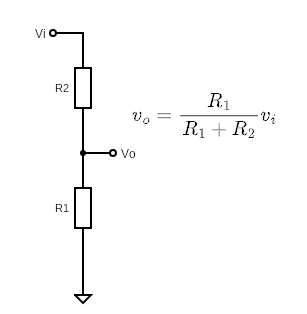top of page# Circuit Essentials - Voltage Divider

Welcome to the first installment of Circuit Essentials, where we'll take a short, mostly qualitative look at some must-know circuits.

Make sure to let us know if you have any circuits or components you'd like to learn more about!

Let’s start easy, and take a quick look at the humble voltage divider.The well-known result on the left informs that the input voltage will drop across each of the resistances in their proportion to the total series resistance (Note the voltage divider also works with impedances as well!). Hence by choosing appropriate relative resistances, the input voltage can be divided at the output to anywhere in the range of 0 to Vi volts.

Great, so can we use a voltage divider any time we want to turn a large voltage into a smaller one? Such as a logic level shifter (5V to 3.3V), or maybe a purely attenuating amplifier? Well, maybe…Voltage dividers are highly subject to the effects of loading. In a circuit, the voltage divider output is connected to other components which load the output with a resistance (or impedance ) RL. Sometimes you can create your voltage divider with the effects of the load in mind, but this may not always be possible. Effects of loading can be minimised by making sure the load resistance is comparatively high to those used in the voltage divider.

But think carefully whether you want to achieve this by lowering the resistances used in the divider, as current, power, and heat will increase! Buffer stages can be a good solution here, or just by making sure the load is sufficiently high impedance.

You’ll start seeing voltage division everywhere, definitely wherever a potentiometer is used, but also creating a voltage bias for components such as transistors and in many other applications.